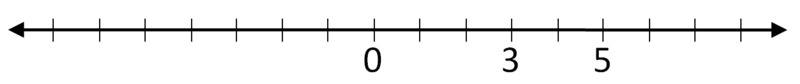# Integers on the Number Line 1

Alignments to Content Standards: 6.NS.C.7.a1. Find and label the numbers $-3$ and $-5$ on the number line.
2. For each of the following, state whether the inequality is true or false. Use the number line diagram to help explain your answers.

1. $-3 \gt -5$
2. $-5 \gt -3$
3. $-5 \lt -3$
4. $-3 \lt -5$

## Solution

1. Because 3 is three units away from 0 (and 5 is five units away from 0), we know that the number line is in increments of 1.

-3 is located three units to the left of zero. We know this because the number 3 tells us how many units from zero the number lies, and the sign of the number tells us which side of zero the number lies. In this case, the sign is negative so we will plot the number to the left of zero.

-5 is located five units to the left of zero. We know this because the number 5 tells us how many units from zero the number lies, and the sign of the number tells us which side of zero the number lies.

Now we can plot -3 and -5.

2.Note: On a number line where positive numbers are to the right of zero and negative numbers are to the left of zero, numbers farther to the right are always greater than those to the left.

$-3 \gt -5$ because -3 is farther to the right than -5.

$-5 \lt -3$ because -5 is farther to the left than -3.

Statements 1 and 3 are true, while statements 2 and 4 are false.